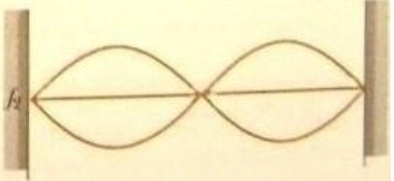# Problem: a) Suppose the tension is quadrupled while the frequency shaking the string is held constant. Will there be a standing wave? If so, draw it. If not, why not?b) Suppose the tension is tripled while the frequency shaking the string is held constant. Will there be a standing wave? If so how many antitodes will it have? If not, why not?

###### FREE Expert Solution

a)

From the diagram, the frequency, f:

$\overline{){\mathbf{f}}{\mathbf{=}}\frac{\mathbf{n}}{\mathbf{2}\mathbf{L}}\sqrt{\frac{\mathbf{T}}{\mathbf{\mu }}}}$

n is the number of loops, in this case, 2.

The velocity of the wave, v:

$\overline{){\mathbf{v}}{\mathbf{=}}\sqrt{\frac{\mathbf{T}}{\mathbf{\mu }}}}$

If the tension is quadrupled,

87% (116 ratings)###### Problem Detailsa) Suppose the tension is quadrupled while the frequency shaking the string is held constant. Will there be a standing wave? If so, draw it. If not, why not?

b) Suppose the tension is tripled while the frequency shaking the string is held constant. Will there be a standing wave? If so how many antitodes will it have? If not, why not?

Frequently Asked Questions

What scientific concept do you need to know in order to solve this problem?

Our tutors have indicated that to solve this problem you will need to apply the Standing Waves concept. You can view video lessons to learn Standing Waves. Or if you need more Standing Waves practice, you can also practice Standing Waves practice problems.

What professor is this problem relevant for?

Based on our data, we think this problem is relevant for Professor Lee's class at Southwestern College.